﻿ 手机惯导与RFID的盲人导航系统设计与实现
«上一篇文章快速检索 高级检索

 智能系统学报2019, Vol. 14Issue (3): 491-499  DOI: 10.11992/tis.2018040580

### 引用本文HUAN Zhan, CHEN Xuejie, LIANG Jiuzhen. Design and implementation of blind-navigation system based on RFID and smartphones’ inertial navigation[J]. CAAI Transactions on Intelligent Systems, 2019, 14(3): 491-499. DOI: 10.11992/tis.201804058.### 文章历史

Design and implementation of blind-navigation system based on RFID and smartphones’ inertial navigation
HUAN Zhan, CHEN Xuejie , LIANG Jiuzhen
School of Information Science and Engineering, Changzhou University, Changzhou 213164, China
Abstract: To help the blind travel independently and conveniently, a design scheme is proposed by combining inertial navigation and radio-frequency identification (RFID) technology with their respective advantages and characteristics. The scheme, which is based on fixed RFID tags, can automatically generate the best route for the blind. With the help of a portable RFID reader installed in personal smartphones, the scheme can accomplish positioning, route planning, and voice prompting. The experimental results show that the system can provide a safe and convenient navigation service for the blind, thus solving their independent-traveling problems.
Key words: RFID tags    inertial navigation    indoor positioning    blind navigation    shortest path    Dijkstra algorithm    restricted rectangle searching area    dynamic time warping

1 系统设计 1.1 RFID选型

1.2 系统结构设计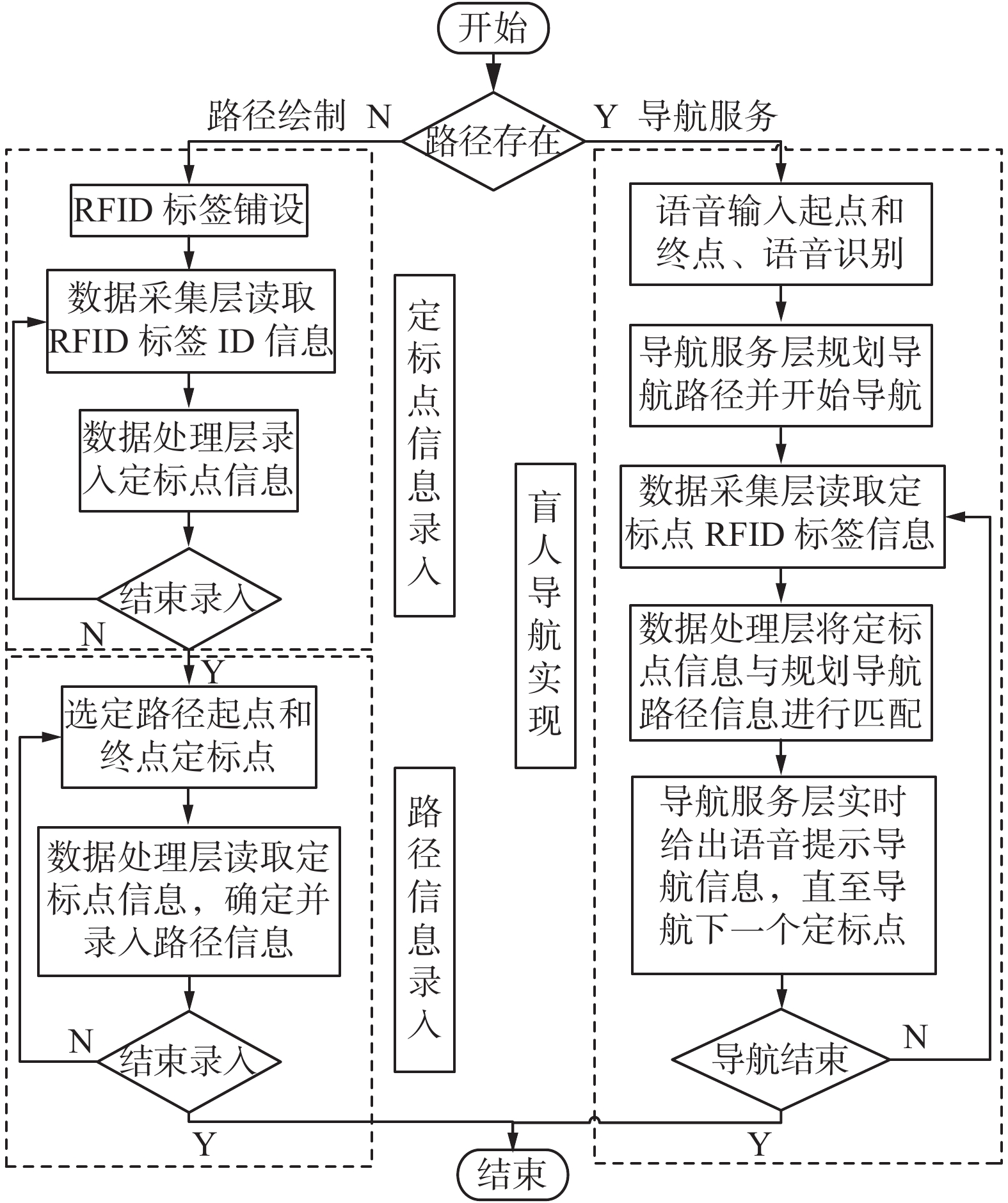Download: 图 1 系统总体流程图 Fig. 1 System overall flow chart
1.3 路径绘制流程设计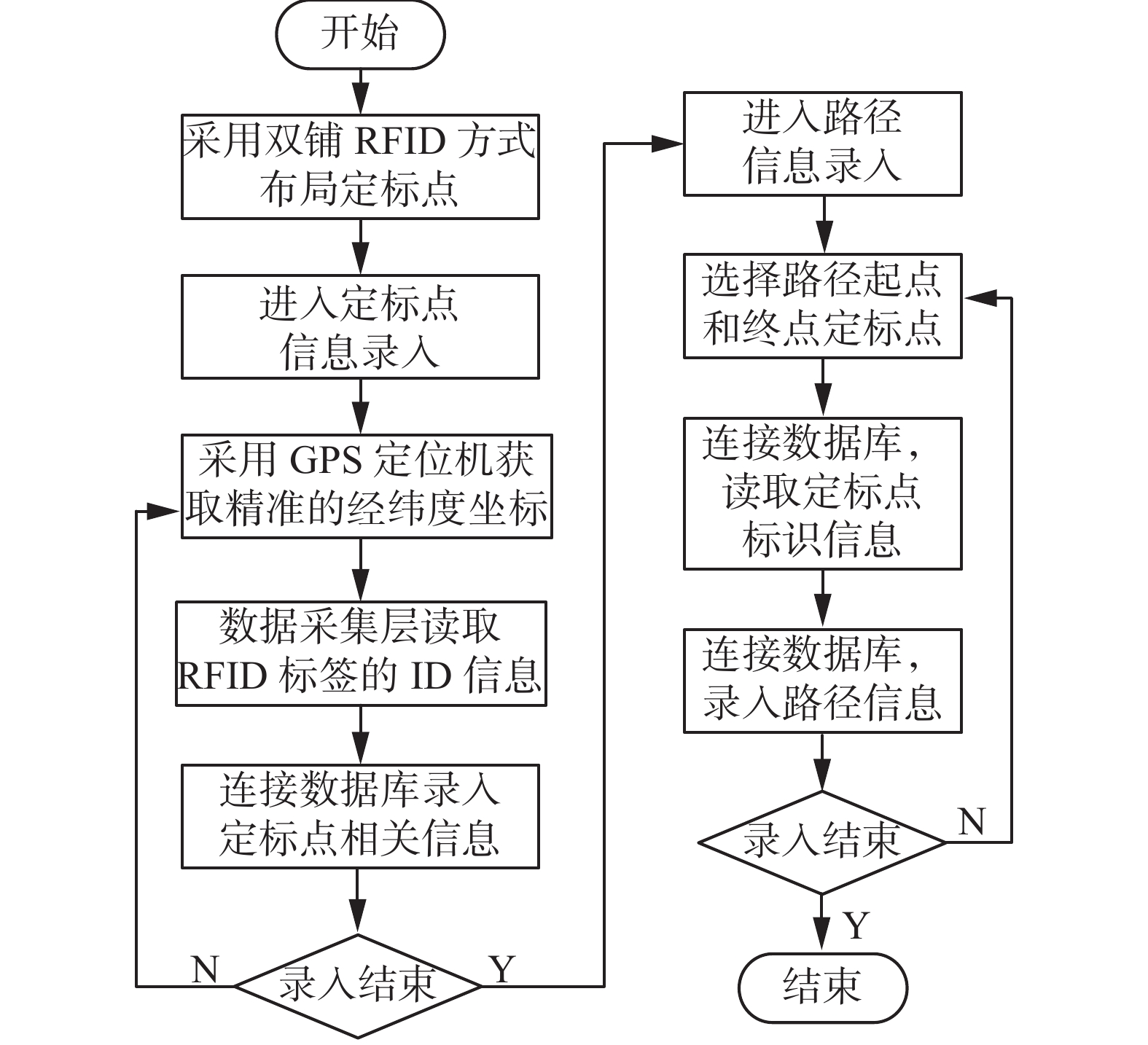Download: 图 2 路径绘制流程图 Fig. 2 Path drawing flow chart
1.4 导航流程设计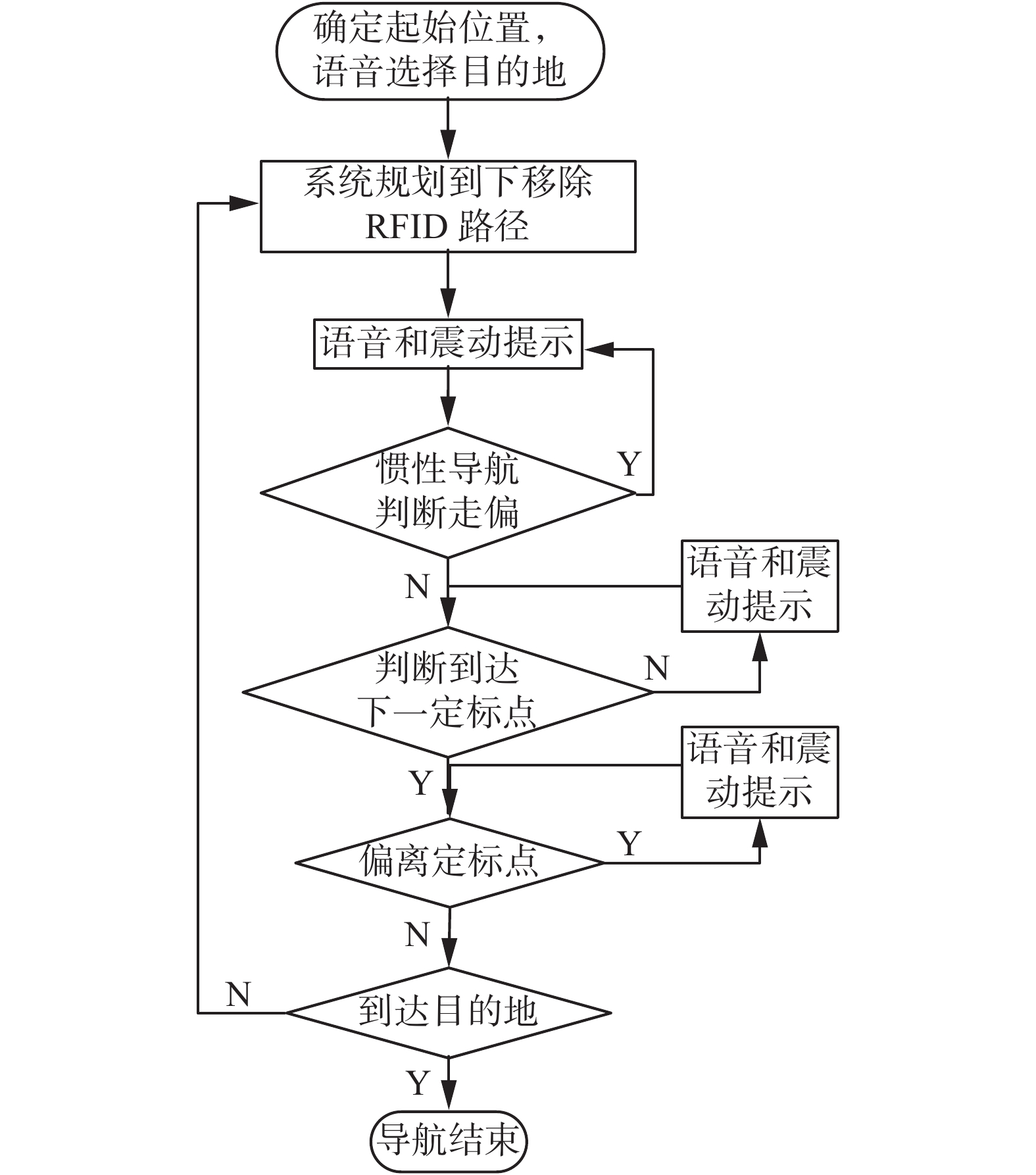Download: 图 3 导航流程图 Fig. 3 Navigational flow chart
1.5 数据库设计表 1 路径信息表(Route_Info) Tab.1 Path information table(Route_Info)表 2 定标点信息表(Label_Info) Tab.2 Fixed punctuation information table
2 系统实现 2.1 数据采集层实现

RFID标签铺设：最常见的RFID铺设方法有单排铺设和双排铺设方法，如图4图5所示。Download: 图 4 单排铺设 Fig. 4 Single row laying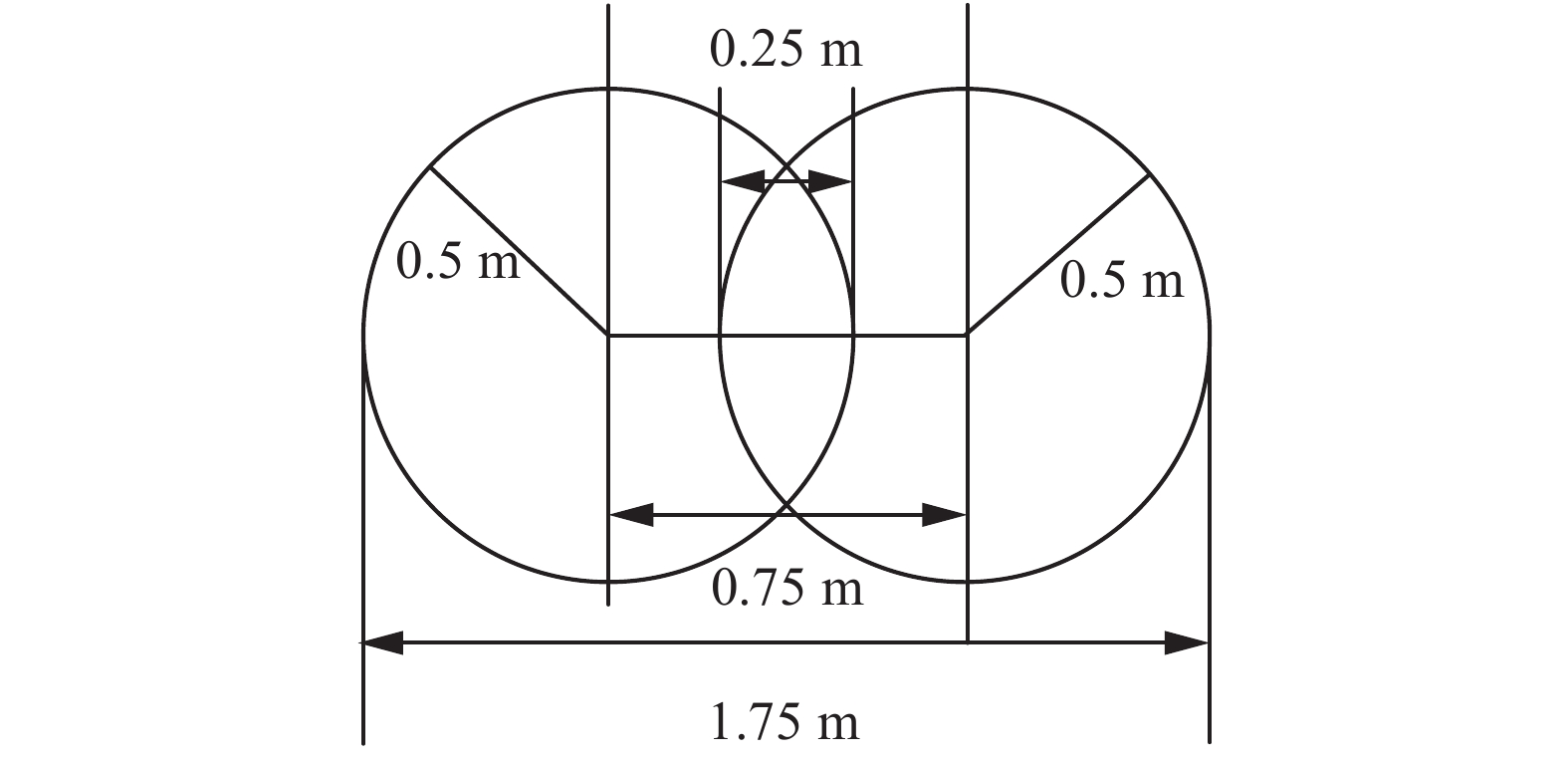Download: 图 5 双排铺设 Fig. 5 Double row laying

2.2 通信传输层实现

2.3 数据处理层实现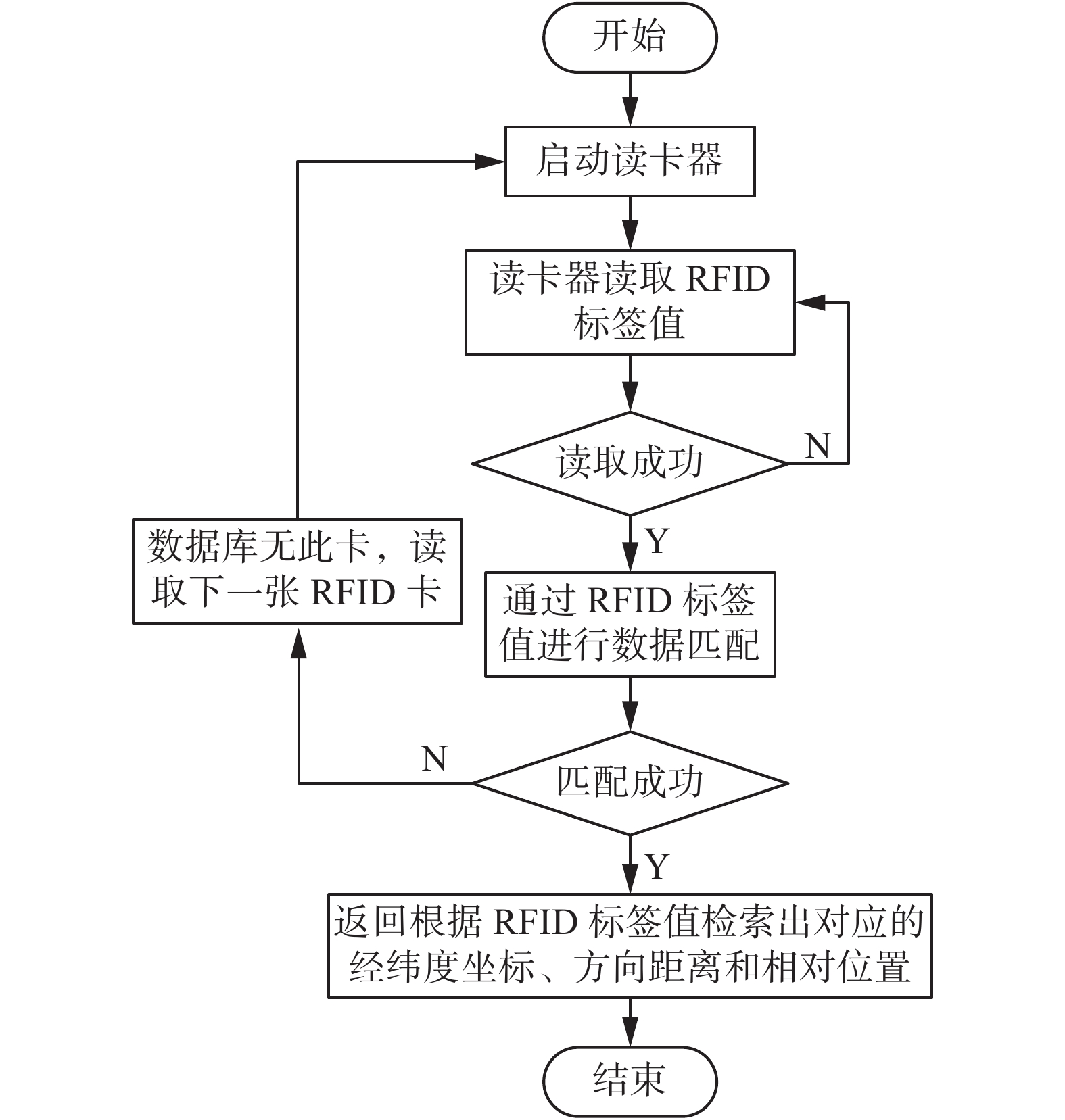Download: 图 6 信息查询流程图 Fig. 6 Information query flow chart
2.4 导航服务层实现 2.4.1 惯性导航

 ${\mathop{\rm SDR}\nolimits} = {\mathop{\rm sd}\nolimits} (T) - \sum\limits_i^{} {\frac{{\left| {{T_i}} \right|}}{{\left| T \right|}}} \times {\mathop{\rm sd}\nolimits} ({T_i})$ (1)

 ${\mathop{\rm LM}\nolimits} ({T_i}) = \sum\limits_{i = 1}^N {({k_i} \times {C_i})}$ (2)

1)标记状态：盲人在监护人的陪同下采集不同行走状态下的加速度数据，系统会给不同状态标记相对应的值。在平时出行过程中盲人一般产生4种运动状态数据，即噪声、静止、步行、上下楼梯，系统分别给它们标记–10、0、10、20。

2)数据预处理：为了达到对加速度数据去噪的目的，系统采用10点的平滑滤波对数据进行预处理。

3)特征提取：提取波峰均值、波峰方差、单步间隔均值、单步间隔方差以及波峰之间的时间间隔方差。

4)模型建立：利用M5模型树根据所选取的特征进行有监督的机器学习，最后输出分类模型。

DTW是一种衡量两个时间序列之间相似度的算法，其算法思想是：令要计算相似度的两个时间列为 XY，长度分别为 $\left| X \right|$ $\left| Y \right|$ ；把路径规整成 $W = {w_1},{w_2},\cdots,{w_k}$ ${\mathop{\rm Max}\nolimits} (\left| X \right|,\left| Y \right|) \leqslant K \leqslant \left| X \right| + \left| Y \right|$ ${w_k}$ 的形式为 $(i,j)$ ，式中 $i$ $j$ 分别为 $X$ $Y$ 中的坐标，规整路径 $W$ 必须从 ${w_1} = (1,1)$ 开始到 ${w_k} = (\left| X \right|,\left| Y \right|)$ 结束。另外， $W$ 中的 $(i,j)$ $i$ $j$ 必须是单调的，即 ${w_k} = (i,j),$ ${w_{k + 1}} = ({i'},{j'}),i \leqslant {i'} \leqslant i + 1,$ $j \leqslant {j'} \leqslant j + 1$ ；最后需要得到最短的一个规整路径， ${\mathop{\rm Dist}\nolimits} (W) = \sum\limits_{k = 1}^{k = K} {{\mathop{\rm Dist}\nolimits} ({w_{ki}},{w_{kj}})}$ ，系统中 ${\mathop{\rm Dist}\nolimits} ({w_{ki}},{w_{kj}})$ 为欧式距离；在实现DTW算法时采用动态规划的思想，其核心见式(3)：

 $\begin{array}{c} D(i,j) = {\mathop{\rm Dist}\nolimits} (i,j) + \min [D(i - 1,j),\\ D(i,j - 1),2D(i - 1,j - 1)] \end{array}$ (3)

1)步长提取：盲人在监护人陪同下采用多次采样取平均的方法采集盲人在正常行走下的步长。

2)数据采集：智能手机采集实时数据，由于统计步数一般需要在实时的状态，采用时间窗口的方式对数据进行采集，每次统计一个时间窗口的步数以及该时间窗口内的方向。

3)数据预处理：与训练模型过程一样，采用10点平滑滤波对数据进行预处理。

4)特征提取与分类输出：提取波峰均值、波峰方差、单步间隔均值、单步间隔方差以及波峰之间的时间间隔方差，将这些特征输入已经建立好的M5分类器中，得到所属分类。

5)计步：使用DTW算法，选择与之相似度最高的状态，如果不是噪声则记为一步，并且记录该步的状态。

6)位置推算：结合步幅的属性、步长以及方向推算出实时位置。

2.4.2 矩形限制区域Dijkstra算法的描述

 $\begin{array}{c} \displaystyle\frac{{{{\left[ {\left( {x - a} \right)\cos \;\varphi + \left( {y - b} \right)\sin \varphi } \right]}^2}}}{{{A^2}}}{\rm{ + }} \\ \displaystyle\frac{{{{\left[ { - \left( {x - a} \right){\rm{sin}}\;\varphi + \left( {y - b} \right){\rm{cos}}\;\varphi } \right]}^2}}}{{{B^2}}}{\rm{ = }}1 \end{array}$ (4)

 ${x_m} = a \pm \sqrt {{A^2}{{\cos }^2}\varphi + {B^2}{{\sin }^2}\varphi }$ (5)
 ${y_m} = b \pm \sqrt {{A^2}{{\sin }^2}\varphi + {B^2}{{\cos }^2}\varphi }$ (6)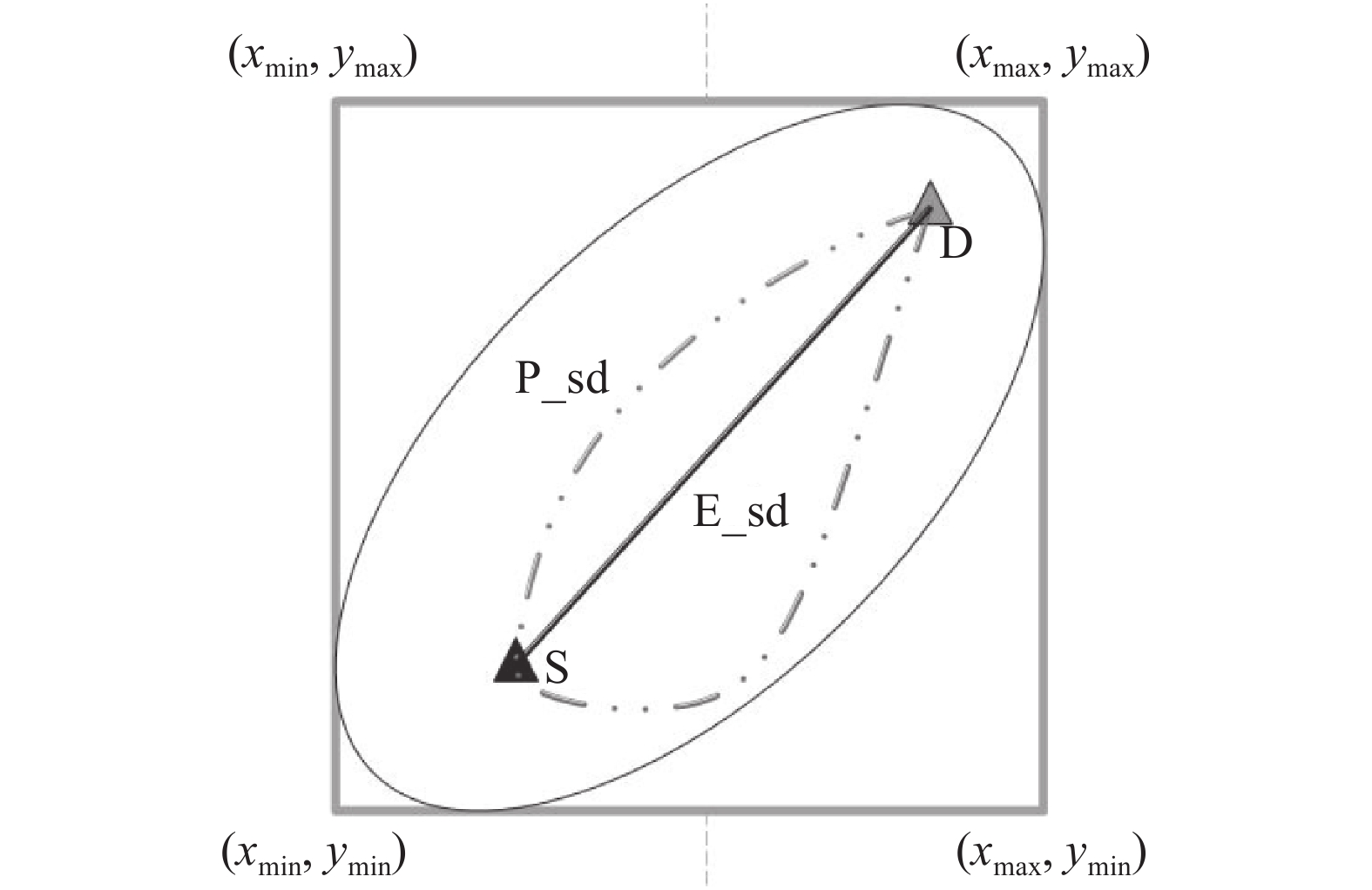Download: 图 7 椭圆及矩形限制搜索区域 Fig. 7 Ellipse and rectangle-restricted search area

1)初始时，C只包含起点，即C＝{S}，v的距离为0。U包含除S外的其他顶点，即U={其余顶点}。若SU中顶点u有边，则<u, S>正常有权值W；若u不是S的邻接点，则<u, S >权值为∞。

2)根据起点 $S({x_s},{y_s})$ 和终点 $D({x_d},{y_d})$ ，计算 $A$ $B$ $a$ $b$ $\varphi$ ，构造椭圆方程，确定椭圆区域。

3)根据已经确定的椭圆方程计算 ${x_{\min }}\text{、}{x_{\max }}\text{、}$ ${y_{\min }}\text{、}{y_{\max }}$ ，确定最后搜索的矩形区域 。

4)从U中选取一个在矩形区域内且权值最小的顶点k，把k加入C中(在初始状态下权值按照式(7)计算)。

5)以k为新考虑的中间点，修改U中各顶点的权值；若从起点S到顶点u的权值(经过顶点k)比原来权值(不经过顶点k)小，则修改顶点u的权值。

6)重复步骤4)、5)，直到找到从SD的一条路径(即所规划路径)。

2.4.3 路径权重初始与更新

 $w = \frac{{{D_{Ai}}}}{{{{\min }_{1 \leqslant i \leqslant k}}({D_{Ak}})}}$ (7)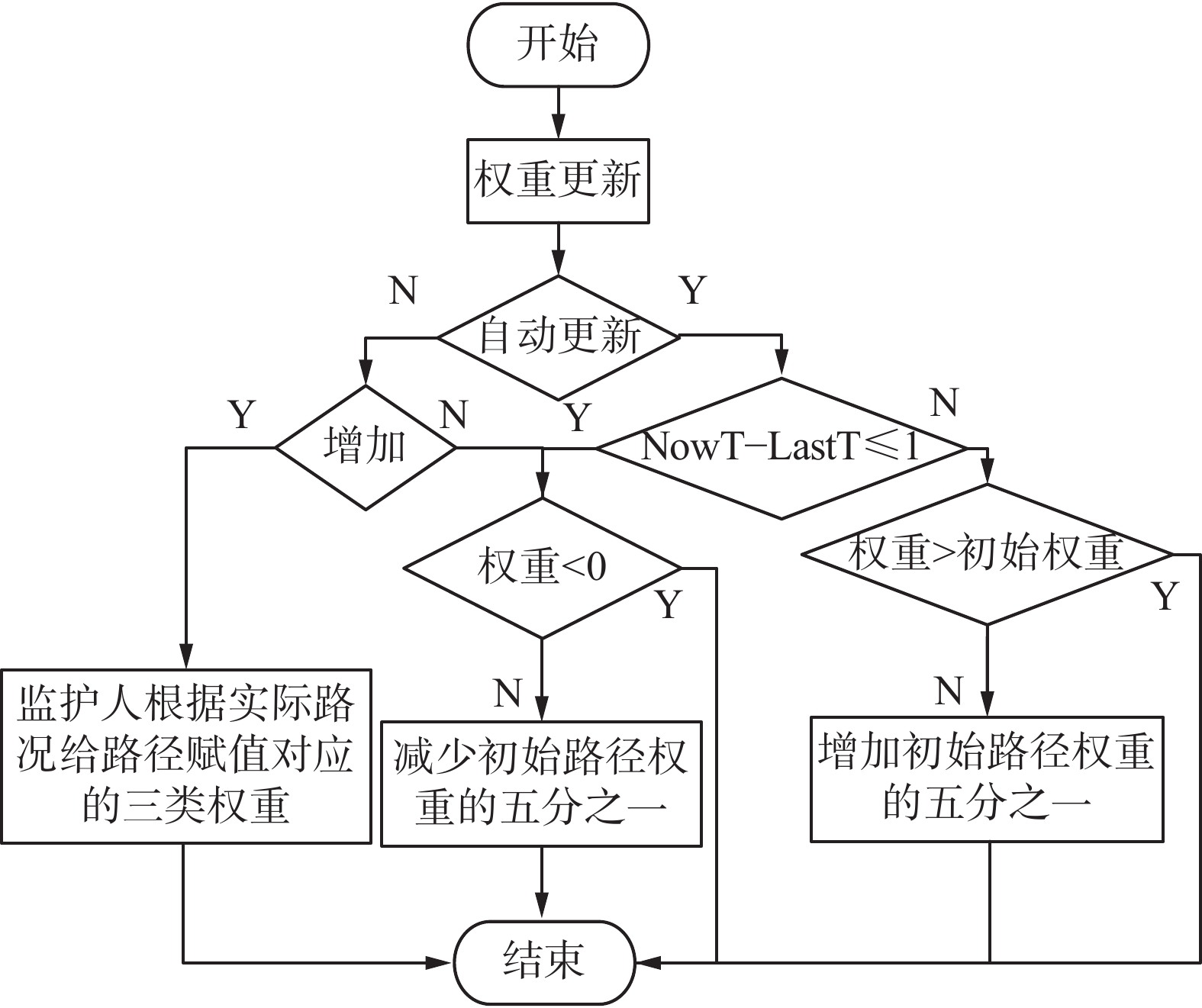Download: 图 8 权重更新算法 Fig. 8 Weight updating algorithm
2.4.4 导航纠偏

2.4.5 语音提示及反馈

3 实验与结果分析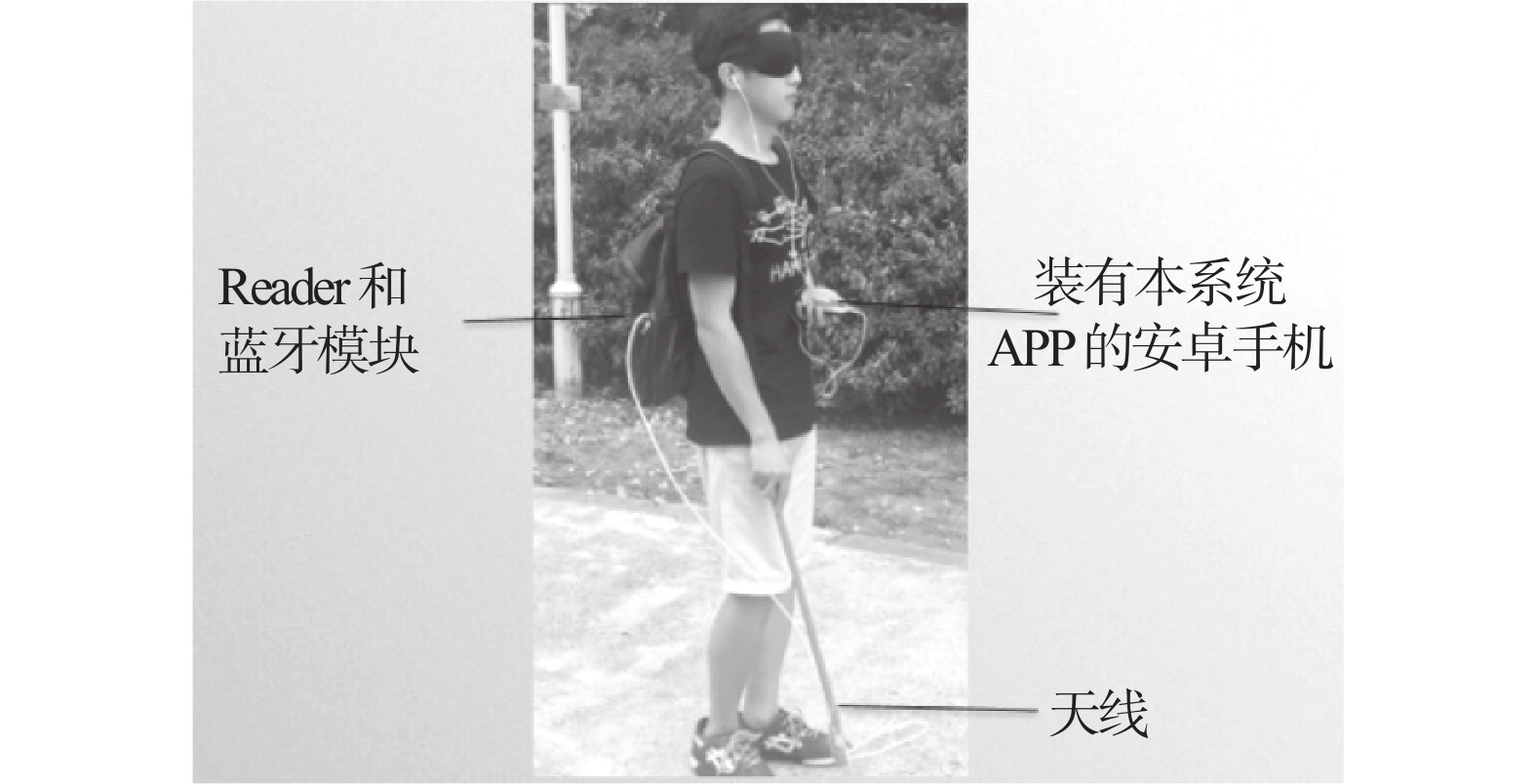Download: 图 9 穿戴效果图 Fig. 9 Wearable effect diagram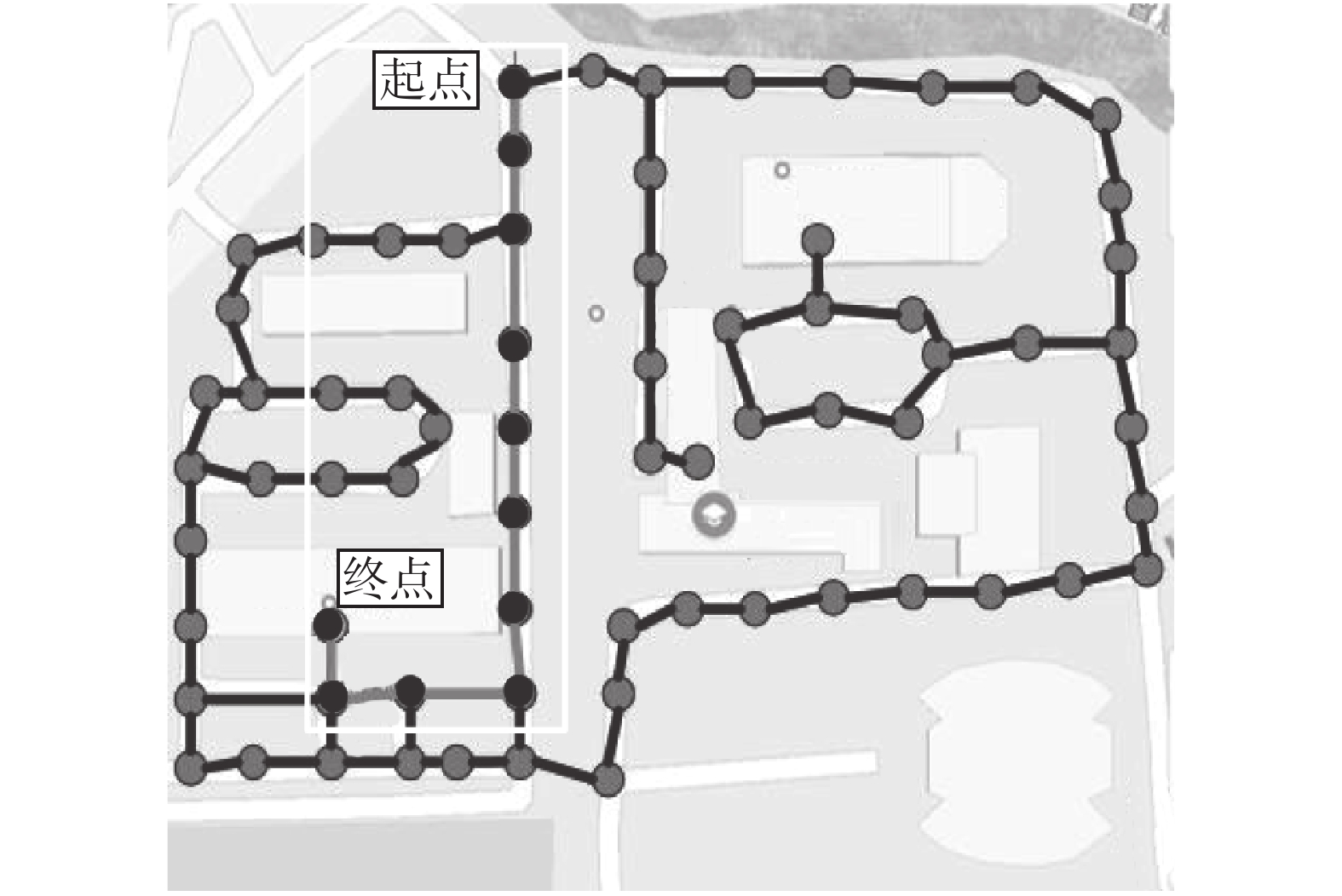Download: 图 10 简单路径效果图 Fig. 10 Simple path effect diagram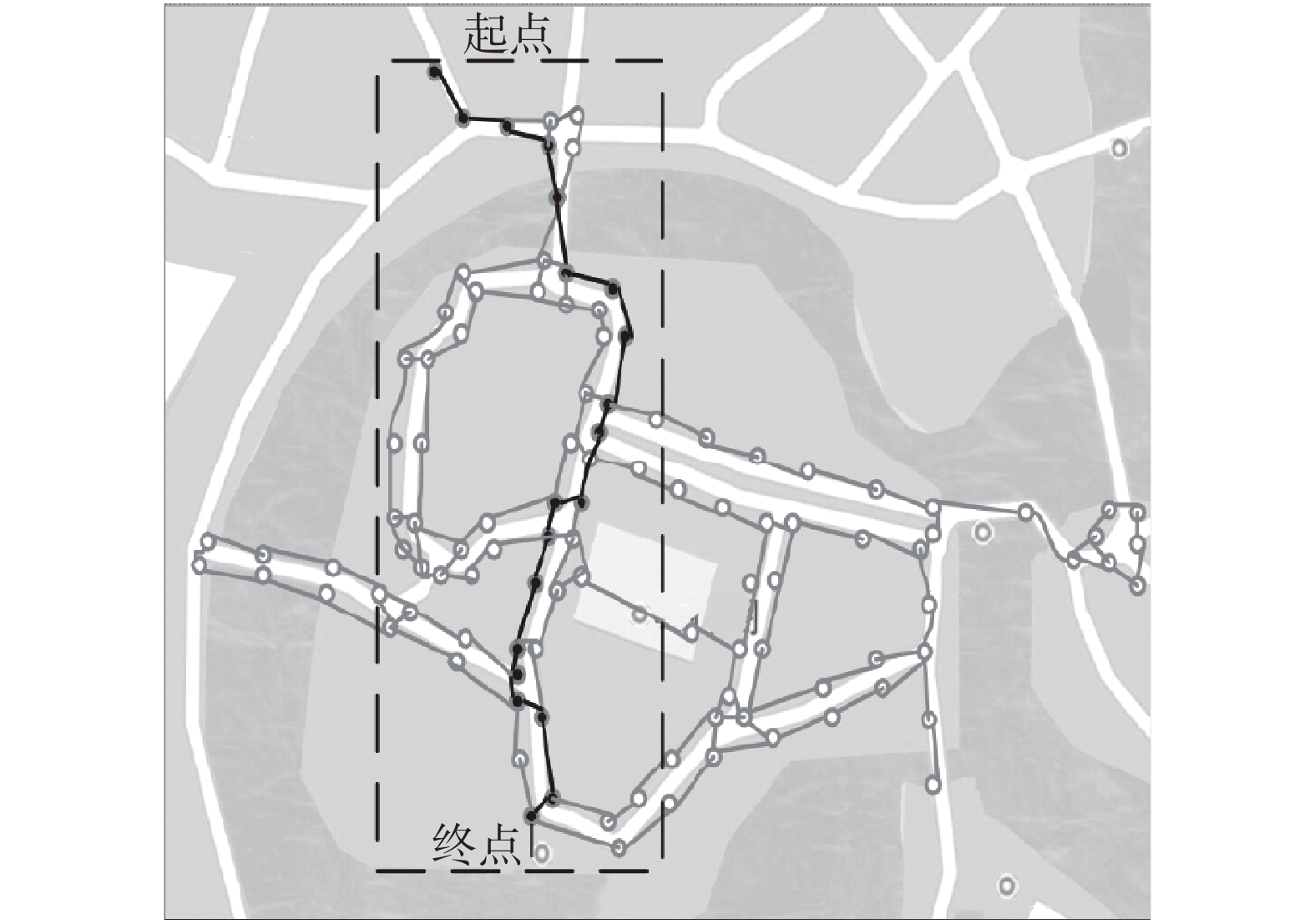Download: 图 11 复杂路径效果图 Fig. 11 Complex path effect diagram表 3 简单路径测试结果 Tab.3 Simple path test results表 4 复杂路径测试结果 Tab.4 Complex path test results

NavCog3利用低功耗蓝牙作为信标点(蓝牙灯塔)，结合惯性导航为盲人自主出行提供导航服务；为了达到更高的精度，蓝牙灯塔之间的间隔为7~10 m，而且为了让无线电波有更好的可到达性，灯塔的高度为2~3.5 m。最终的实验结果表明：NavCog3的平均导航误差为1.65 m。相对于NavCog3，系统采用纯RFID标签路径布局方式(每隔20 m布局一组)RFID标签，1 km需要100个RFID标签，每个标签的成本为0.3元，1 km的成本为30元)，成本低廉利于大范围推广，并且平均导航误差为0.467 9 m优越于NavCog3。

4 结束语

  CAO Lin, HUANG Xiaoli. GPS-based navigation for the blind[J]. Advanced materials research, 2012, 433–440: 4184-4188. (0)  崔金琦, 陶先平. 基于RFID的校园导航系统的设计与实现[J]. 计算机科学, 2015, 42(12): 92-94,119. CUI Jinqi, TAO Xianping. Design and implementation of RFID-based campus navigation system[J]. Computer science, 2015, 42(12): 92-94,119. (0)  谢磊, 殷亚凤, 陈曦, 等. RFID数据管理: 算法、协议与性能评测[J]. 计算机学报, 2013, 36(3): 457-470. XIE Lei, YIN Yafeng, CHEN Xi, et al. RFID data management: algorithms, protocols and performance evaluation[J]. Chinese journal of computers, 2013, 36(3): 457-470. (0)  曹敏. 基于DSP的高频远距离RFID读写器的研究与实现[D]. 济南:山东大学, 2013. CAO Min. The research and implementation of the long range HF RFID reader based on DSP[D]. Ji’nan: Shandong University, 2013. (0)  张文杰. 基于RFID和地磁场联合的室内定位技术研究[D]. 南京:南京邮电大学, 2015. ZHANG Wenjie. Research on hybrid indoor positioning with RFID and magnetic field[D]. Nanjing: Nanjing University of Posts and Telecommunications, 2015. (0)  高佩, 刘洪星, 张朋, 等. 基于Android平台的盲人手机系统设计与开发[J]. 电脑知识与技术, 2014, 10(19): 4447-4449, 4459. GAO Pei, LIU Hongxing, ZHANG Peng, et al. Design and implementation of mobile phone system for the blind based on android[J]. Computer knowledge and technology, 2014, 10(19): 4447-4449, 4459. (0)  万骏炜, 曾庆化, 陈磊江, 等. 行人惯性导航系统平台设计与实现[J]. 计算机应用与软件, 2015, 32(2): 45-47, 170. WAN Junwei, ZENG Qinghua, CHEN Leijiang, et al. Design and implementation of pedestrian inertial navigation system[J]. Computer applications and software, 2015, 32(2): 45-47, 170. DOI:10.3969/j.issn.1000-386x.2015.02.011 (0)  ERIC S. Navigation system for blind pedestrians and public transport users[J]. I-com, 2014, 13(3): 12-18. (0)  BARATI F, DELAVAR M R. Design and development of a mobile sensor based the blind assistance wayfinding system[J]. International archives of the photogrammetry, remote sensing and spatial information sciences, 2015, XL–1(W5): 91-96. (0)  聂元祥. 多功能导航盲杖[P]. 中国: 203425209, 2014–02–12. (0)  叶海波. 基于智能手机的室内标签定位技术研究[D]. 南京:南京大学, 2016. YE Haibo. Smartphone based indoor localization for symbolic position[D]. Nanjing: Nanjing University, 2016. (0)  邢珍珍. 基于地磁传感器的室内导航解决方案[J]. 软件工程, 2016, 19(4): 13-15. XING Zhenzhen. Indoor navigation solution based on geomagnetic sensor[J]. Software engineering, 2016, 19(4): 13-15. DOI:10.3969/j.issn.1008-0775.2016.04.005 (0)  范荣双, 王继周, 房慎冲, 等. 室内地磁导航基准图生成方法及系统[P]. 中国: 104807462, 2015-07-29. (0)  郭才发, 胡正东, 张士峰, 等. 地磁导航综述[J]. 宇航学报, 2009, 30(4): 1314-1319, 1389. GUO Caifa, HU Zhengdong, ZHANG Shifeng, et al. A survey of geomagnetic navigation[J]. Journal of astronautics, 2009, 30(4): 1314-1319, 1389. DOI:10.3873/j.issn.1000-1328.2009.04.002 (0)  GALLAGHER T, WISE E, YAM H C, et al. Indoor navigation for people who are blind or vision impaired: where are we and where are we going?[J]. Journal of location based services, 2014, 8(1): 54-73. DOI:10.1080/17489725.2014.895062 (0)  RIEHLE T H, ANDERSON S M, LICHTER P A, et al. Indoor magnetic navigation for the blind[C]//Proceedings of Annual International Conference of the IEEE Engineering in Medicine and Biology Society. San Diego, CA, USA, 2012: 1972. (0)  徐向军. 惯性测量与航迹推算的室内定位方法[D]. 无锡:江南大学, 2016. XU Xiangjun. The indoor location method based on inertial measurement and dead reckoning[D]. Wuxi: Jiangnan University, 2016. (0)  周婧, 陈庙红, 吴豪杰. 基于惯性导航的平面航迹推算的研究[J]. 计算机科学, 2017, 44(6A): 582-586. ZHOU Jing, CHEN Miaohong, WU Haojie. Research on plane dead reckoning based on inertial navigation system[J]. Computer science, 2017, 44(6A): 582-586. DOI:10.11896/j.issn.1002-137X.2017.6A.131 (0)  徐鼎, 孟坤, 李尚同. 基于Android端的惯性导航算法研究[J]. 软件导刊, 2017, 16(4): 35-37. XU Ding, MENG Kun, LI Shangtong. Research on inertial navigation algorithm based on Android[J]. Software guide, 2017, 16(4): 35-37. (0)  黄政, 韩立新, 肖艳. 基于M5和DTW的计步方法设计[J]. 计算机学报, 2017, 40(08): 1856-1871. HUANG Zheng, HAN Lixin, XIAO Yan. Step detection design approach based on M5 and DTW[J]. Chinese journal of computers, 2017, 40(08): 1856-1871. (0)  王海梅, 周献中. 一种限制搜索区域的最短路径改进算法[J]. 南京理工大学学报 (自然科学版), 2009, 33(5): 638-642. WANG Haimei, ZHOU Xianzhong. Improved shortest path algorithm for restricted searching area[J]. Journal of Nanjing University of Science and Technology (natural science), 2009, 33(5): 638-642. (0)  王亚文, 汪西莉, 曹菡, 等. 一种动态限制搜索区域的最短路径规划算法[J]. 计算机应用研究, 2007, 24(7): 89-91. WANG Yawen, WANG Xili, CAO Han, et al.. Shortest route planning algorithm within dynamic restricted searching area[J]. Application research of computers, 2007, 24(7): 89-91. DOI:10.3969/j.issn.1001-3695.2007.07.028 (0)  石婷. 基于Android的LBS应用研究[D]. 西安:西安电子科技大学, 2014. SHI Ting. Research on application of LBS based on Android[D]. Xi’an: Xidian University, 2014. (0)  SATO D, OH U, NAITO K, et al. NavCog3: an evaluation of a smartphone-based blind indoor navigation assistant with semantic features in a large-scale environment[C]//The International ACM Sig Access Conference. Baltimore, MD, USA, 2017: 270-279. (0)